Congruence Subgroups of SL2(Z)

Math 252: Modular Abelian Varieties

William Stein

How to conjugating an element of the upper half plane into the fundamental domain for PSL2(Z)

Let D be the fundamental domain for G=PSL2(Z) that I described in the last lecture, which is illustrated below: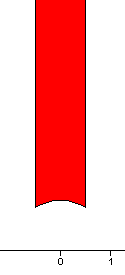Last time we proved that if z is any point in the upper half plane, then there is an element g in G such that g(z) is in D. The proof was nonconstructive. Here is an algorithm to find such a g in practice.

 Do the following until z is in D:       1. Replace z by z+n where n is an       integer such that |Re(z+n)| <= 1/2.    2. If |z|<1, replace z by -1/z.

This process must terminate, because in step 2 the imaginary part of -1/z is bigger than the imaginary part of z, and the proof from last time showed that the imaginary parts of the conjugates of z are bounded above.

Remarks.

1. John Cremona makes the following remark when describing this algorithm in Section 2.14 of his book. "In practice one must be careful about rounding errors, as it is quite possible to have both |z|<1 and |-1/z|<1 after rounding, which is liable to prevent the algorithm from terminating." So be careful if you implement this algorithm.
2. This is almost the same as the algorithm for computing the continued fraction expansion of a real number! Explore this further on your own if you want.

Writing an element in terms of generators

Note that this algorithm also gives an algorithm to write an arbitrary element of G in terms of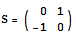and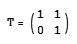.

For example, suppose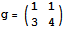. Hit 2i by g, then find a combination of S and T that conjugates g back into D. We get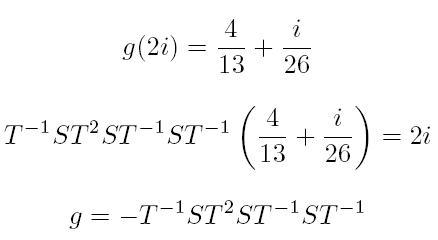Remark. If you wanted to do computations like this efficiently, you would obtain the representation of g from the partial convergents of the continued fraction of a/b, where a and b are the entries in the first column of g. See the end of Section 2.1 of Cremona's book for enough of a hint as to what to do.

Congruence Subgroups

As you will show in your homework reduction modulo N is surjective, so we have an exact sequence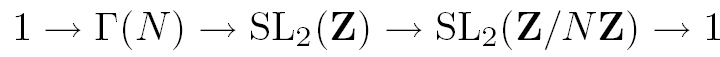DEFINITION: The group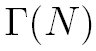is by definition the kernel of the reduction map. It is called the principal congruence subgroup of level N. A congruence subgroup is any subgroup of SL2(Z) that containsfor some N.

It is clear that any congruence subgroup has finite index, sincedoes. What about the converse? This is the so-called "congruence subgroup problem." According to this MathSciNet review, if p is a prime, then every finite index subgroup of SL2(Z[1/p]) is a congruence subgroup, and for any n>2, all finite index subgroups of SL2(Z) are congruence subgroups. However, there are plenty of finite index subgroups of SL2(Z) that are not congruence subgroups. This paper by Hsu contains an algorithm to decide if a finite index subgroup is a congruence subgroup, and gives an example of a subgroup of index 12 that it is not a congruence subgroup. Also this MathSciNet review of a paper by Thompson is about his proof of a surprising conjecture of Atkin about modular forms for noncongruence subgroups.

Sinceis a kernel, it is a normal subgroup. There are two other extremely popular families of congruence subgroups, neither of which are normal: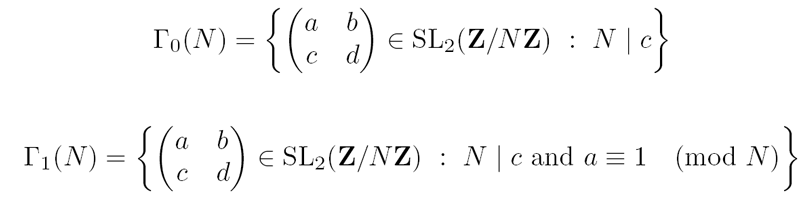Drawing Fundamental Domains for Congruence Subgroups

Helena Verrill's fundamental domains program draws fundamental domains. You should probably look through her description of the interesting mathematical algorithms used in the program. Here are three screen shots, which illustrates some fundamental domains.

Gamma(3)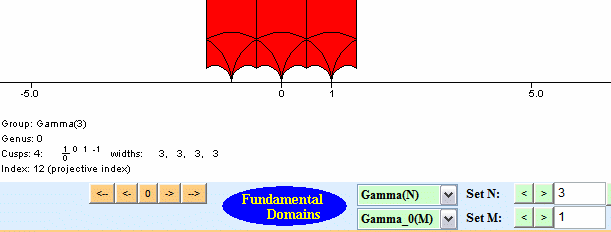Gamma1(3)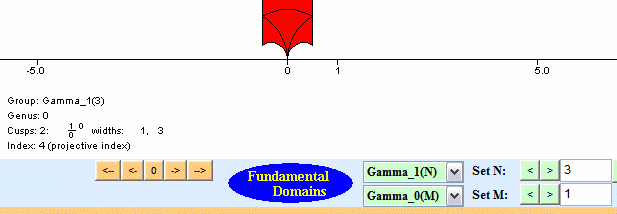Gamma0(11)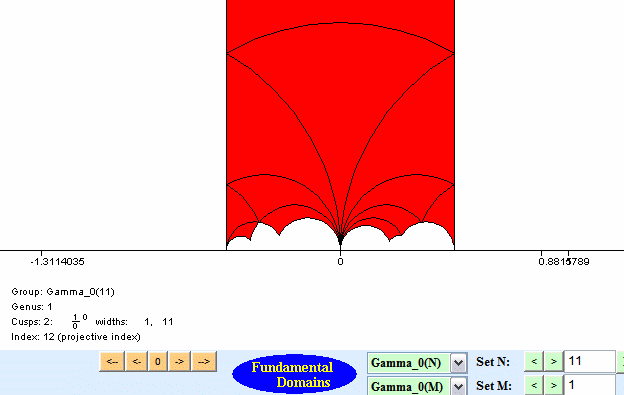The program works by finding right coset representatives for the congruence subgroup in SL2(Z), and translating around a fundamental domain for SL2(Z). The coset representatives are chosen, as described in Verrrill's paper, so that the domain is connected and not tiny -- it wouldn't be interesting to draw a fundamental that consists of a few dots on the screen, even though it might be accurate!

Coset Representatives

Finding coset representatives for any of the three families of congruence subgroups mentioned above is relatively straightforward. To make the problem easy to think about, note that you can quotient out byfirst. Then the question amounts to finding coset representatives for a subgroup of SL2(Z), which is reasonably straightforward. For example:

Proposition. The coset representatives for Gamma_0(N) are in natural bijection with the elements of P1(Z/NZ).

Generators for Congruence Subgroups

Simple Algorithm

Let G be a finite index subgroup of SL2(Z). Let R be a set of coset representatives for G. Define maps s and t from R to G as follows. If r in R then there exists a unique r' in R such that GrS = Gr'. Let s(r) = rS(r')^(-1). Likewise, there is a unique r' such that GrT = Gr' and we let t(r) = rT(r')^(-1). Note that s(r) and t(r) are in G for all r.

Proposition. G is generated by the union of s(R) and t(R).

Proof. Omited.

Sophisticated Algorithms

There are much more sophisticated algorithms that use group actions on trees. Verrill implemented much of this in MAGMA, but unfortunately I don't have time to talk about it today.

Next Week: Modular Curves

Next week we will consider the quotient of the upper half plane by the action of a congruence subgroup. This quotient space is a noncompact Riemann surface. The missing cusps are in bijection with the orbits of the action of the congruence subgroup on P1(Q). Adding them in we obtain a compact Riemann surface. On Monday we'll construct this Riemann surface, and discuss some of its properties. On Wednesday, we'll talk about how to represent its homology using modular symbols. On Friday, we'll describe a finite presentation for homology that is due to Yuri Manin, and sketch his proof that the presentation is correct.